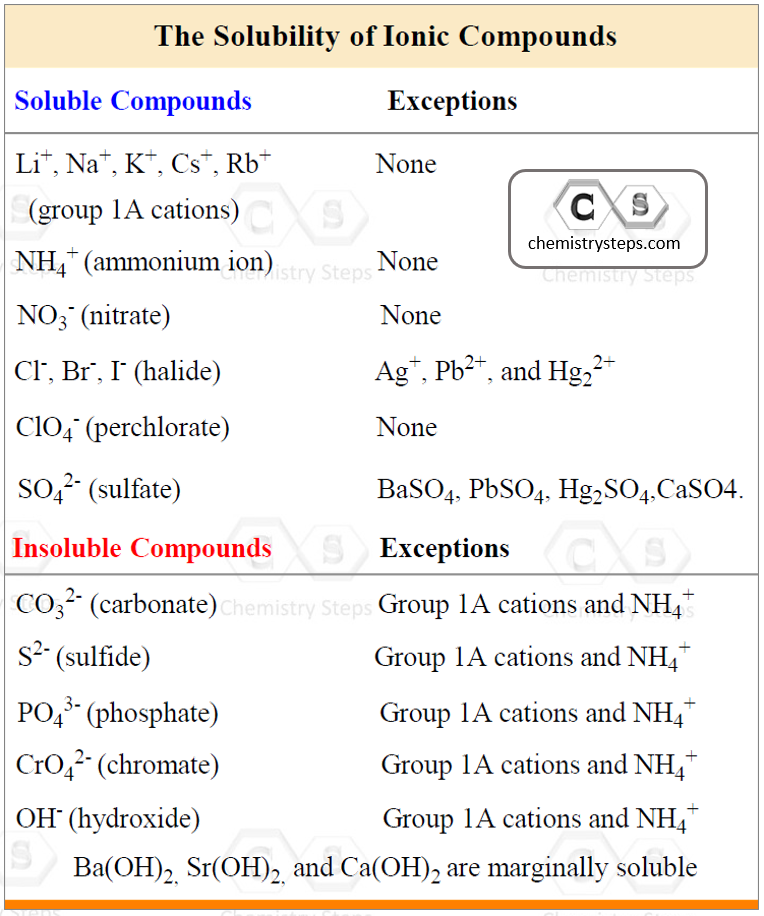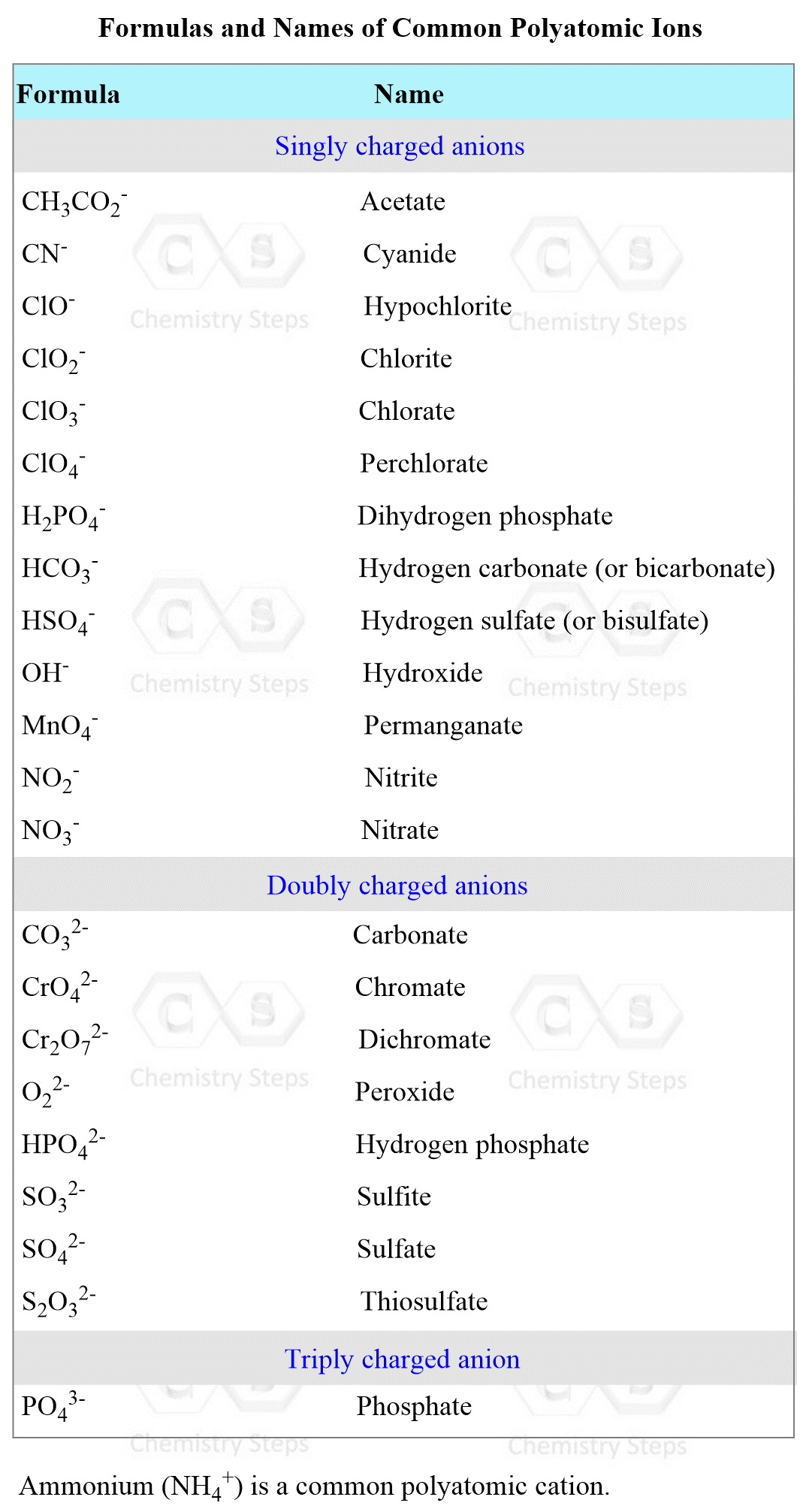## General Chemistry

Water-soluble ionic compounds such as acids, bases, and salts dissociate in water forming ions.

For example, sodium chloride (NaCl) dissociates into Na+ and Cl ions when dissolved in water:

NaCl(aq)  →  Na+(aq) + Cl(aq)

The “aq” notation indicates that the salt is water-soluble, and the ions produced by dissociation/ionization of the salt are surrounded by water molecules. In fact, this interaction between the ions and water molecules is the driving force for dissolving and ionizing the salt.

# Determining the Solubility

The first thing you will need is to determine whether the compound is soluble or not. If it is not, then you cannot write a dissociation equation for it because it only dissociates when dissolved in water.

To determine this, use the following rules for different combinations of cations and anions that make the salt:Using the information in the table, let’s determine, for example, if Ca(ClO4)2 is soluble or not. The perchlorate ion (ClO4) is listed as soluble with any cation without exceptions. Therefore, it is a soluble salt. This, in turn, indicates that Ca(ClO4)is going to dissociate into ions because it is an ionic compound:

Ca(ClO4)2(aq)  →  Ca2+(aq)  +  2ClO4(aq)

Again, do not dissociate a compound that is insoluble!

For example, the sulfate ion (SO42-) is listed under the soluble compounds which means most of its salts and acids are soluble. However, under the exceptions, we see that BaSO4, for example, is insoluble, and therefore, it does not dissociate in water.

# How to write dissociation equations

Once you found out that the compound is water-soluble, you need to write a chemical equation separating the ions.

The key part here is recognizing the ions. You must know the formula and the charge for each ion in the salt in order to avoid having wrong formulas by using omitting or adding the subscripts and charges in the formula.

Here is one typical example of a wrong dissociation equation:

BaBr2(aq)  →  Ba2+(aq)  +  Br2(aq) Wrong!

You can try to figure out what is wrong in the equation, and we will get back to it once we go over the ions you will need to know.

Some of them are the “good guys” as we can easily figure out their charges. For example, the following ions in groups 1A, 2A, and 7A as their charges are predictable and go based on their group:And this table should already point out the mistake in the dissociation of BaBr2. The formula of bromide ion is Br, and that is how it must be written in the dissociation equation of any compound regardless if the bromine has a subscript in it or not.

NaBr(aq)  →  Na+(aq)  +  Br(aq)

BaBr2(aq)  →  Ba2+(aq)  +  2Br(aq)

AlBr3(aq)  →  Al3+(aq)  +  3Br(aq)

The formula of bromide ion is Br, and that is how it

must be written in the dissociation equation

So, do not just carry the subscript to the other side of the equation, and do not balance the equation by adding or removing any subscript.

We do need to carry the subscript if it is part of the formula.

For example, let’s write the dissociation equation of Al(NO)3 step-by-step.

Step 1) Identify the ions; the salt consists of Al3+ and NO3 (nitrate) ions and this means the oxygen is going to have the subscript 3:

Step 2) Break down the salt into its ions Al3+ and NO3:

Al(NO)3(aq)  →  Al3+(aq)  +  NO3(aq)

Step 3) Balance the dissociation equation by adding coefficients (do not change subscripts):

Al(NO)3(aq)  →  Al3+(aq)  +  3NO3(aq)

Use this strategy to dissociate any water-soluble compound. The formulas of the most common polyatomic ions are given below as well:Check Also

#### Practice

1.

Provide a balanced chemical equation for the dissociation of each compound:

a) Na3PO4,  b) K2CrO4,  c) MgCl2,  d) Al(NO3)3,  e) HNO3,  f) K2SO4,  g) Ca(NO2)2,  h) Mg(C2H3O2)2,  i) Na2CO3,  j) (NH4)2SO4,  k) HClO4,  l) NaHSO3,

a)
This content is available to registered users only. Click here to Register! By joining Chemistry Steps, you will gain instant access to the answers and solutions for all the Practice Problems and the powerful set of General Chemistry 1 and 2 Summary Study Guides.
b)
This content is available to registered users only. Click here to Register! By joining Chemistry Steps, you will gain instant access to the answers and solutions for all the Practice Problems and the powerful set of General Chemistry 1 and 2 Summary Study Guides.
c)
This content is available to registered users only. Click here to Register! By joining Chemistry Steps, you will gain instant access to the answers and solutions for all the Practice Problems and the powerful set of General Chemistry 1 and 2 Summary Study Guides.
d)
This content is available to registered users only. Click here to Register! By joining Chemistry Steps, you will gain instant access to the answers and solutions for all the Practice Problems and the powerful set of General Chemistry 1 and 2 Summary Study Guides.
e)
This content is available to registered users only. Click here to Register! By joining Chemistry Steps, you will gain instant access to the answers and solutions for all the Practice Problems and the powerful set of General Chemistry 1 and 2 Summary Study Guides.
f)
This content is available to registered users only. Click here to Register! By joining Chemistry Steps, you will gain instant access to the answers and solutions for all the Practice Problems and the powerful set of General Chemistry 1 and 2 Summary Study Guides.
g)
This content is available to registered users only. Click here to Register! By joining Chemistry Steps, you will gain instant access to the answers and solutions for all the Practice Problems and the powerful set of General Chemistry 1 and 2 Summary Study Guides.
h)